Maths-
General
Easy

Question

# On a certain sum of money, the difference between the compound interest for a year payable half-yearly and the simple interest for a year is  16. Find the sum lent out; if the rate of interest in both cases is 8%.Hint:

## The correct answer is: 10000 Rupees

### Complete step by step solution:Let the sum of money = PIt is given that rate of interest R = 8% and number of years T = 1Also Compound interest - Simple interest = CI - SI  = Rs 16We calculate simple interest by the formula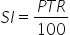…(i)where P is Principal amount, T is number of years and R is rate of interestHere, we have T = 1 years, R = 8%  and P = ?On substituting the known values in (i), we get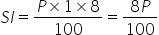…(ii)We calculate compound interest by the formula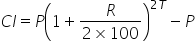…(iii) payable half yearlywhere P is Principal amount, T is number of years and R is rate of interestHere, we have T = 1,R = 8% and P = ?On substituting the known values in (iii), we get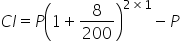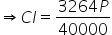…(iv)We have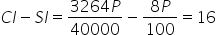On further simplifications, we have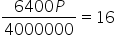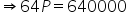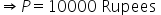Hence the sum of money P = 10000 Rupees.#### With Turito Foundation.#### Get an Expert Advice From Turito.